Pelton

Probably the Pelton  turbine is the only impulse turbine universally used nowadays. In (fig. 1) there is a schematized Pelton bucket with highlighted the most important geometrical quantities. Particular attention has to be put on the opening E at the bucket end, in correspondence to the external diameter of the same, which width shall be slightly bigger than that of nozzle diameter. This opening has the double purpose to allow the jet to operate for a longer time on the bucket without it is disturbed by the arrival of the following bucket.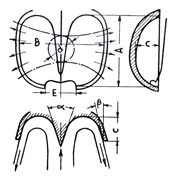fig. 1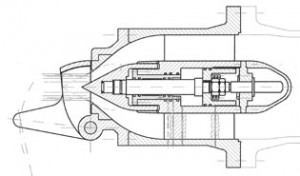fig. 2 Injector

The edges of the opening must be particularly sharpened in order to limit as much as possible the unavoidable water sprays to any direction when they enter in touch with the jet.
These sprays are to the detriment of the efficiency and of the regular operation of the machine. The speed of the water that comes from the nozzle and then strikes the bucket is: ${V}_{ 1 }=\sqrt { 2gH }$  

One of the most significant values for dimensioning one Pelton wheel is the peripheral speed coefficient that is defined by K=U/V  where as usual U is the peripheral speed of the wheel calculated on the pitch diameter D of the nozzle (fig. 3). The maximum value K can assume is 0.485 (to demonstrate this value is beyond the purpose of this site). Anyway, the values usually adopted may vary from 0.45 to 0.485 (see example)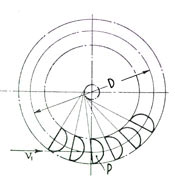fig 3 Pitch diameter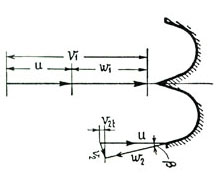fig 4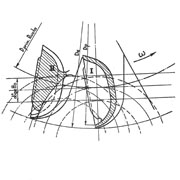fig 5 Relative nozzle

D/d Ratio and number of nozzles
Said d the diameter of the jet coming out from the nozzle (fig.2) and as usual Q the discharge, we can write for a single jet turbine $\bf Q=\frac { {\large V }_{\large 1 }\Large\pi {\large d }^{ 2 } }{\large 4 }$ or  $\bf d=\sqrt {\frac {\large 4Q}{\Large\pi {\large V}_{ 1 }}}$ 

For obvious reasons (fig. 3) it is clear that the ratio D/d cannot reach too small values. In general the limit adopted is D/d>7 even if in extreme conditions it is possible to go down to smaller values. The ratio D/d is modified by increasing the number of nozzles because the discharge shall be subdivided among them and then the diameter of nozzle d will decrease.

Deflector
Pelton turbines, besides the needles that are controlled by the governor that fixes the opening in accordance with the power requested at the moment, have a device called deflector (fig 6 and 7) that has the main task to deflect the jet in a very short time, 1÷2 seconds in case there is a load rejection. The deflected jet does not act any more on the wheel and allows the machine to remain within a limited number of revolutions and in this way the unit shall not be brought to runaway speed, generally 1.8 times the rated speed with remarkable stress for the devices under operation, because of lack of resisting torque with respect to the motor torque, and this gives rise to remarkable stress on the rotation devices.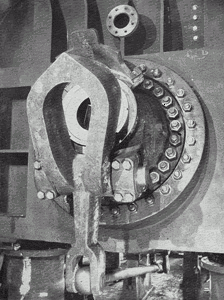fig 6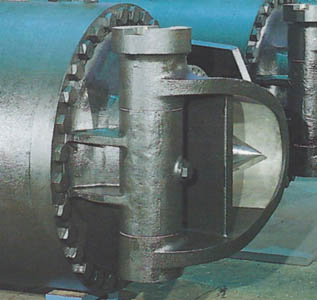fig 7

Dimensions of Pelton wheel buckets
Dimension B (fig. 1) may assume on average the value B = (2.8 ÷ 3.5)d depending on the different manufacturers. For nozzles exceeding 200 mm we shall adopt values smaller than B while for d<20 mm we can adopt B= (4 ÷ 5)d. Making reference again to (fig. 1), we can assume:

A= (0,8 ÷ 0,9)B;   C= (0,25 ÷ 0,3)B;    E=d+(10 ÷ 40)

Angle $\bf\alpha$ is generally included between 20°÷30°. The outlet angle $\bf\beta$ will have great importance because it directly affects the outlet absolute speed V2, (fig 4)that we will try to render as small as possible in order to decrease the percentage of lost drop due to it, that is  $H=\frac { {\large V }_{ 2 }^{ 2 } }{\large 2g }$

Another not less important bond this angle $\bf\beta$ shall have to respect is given by the fact that the water going out from one bucket must not hit the front bucket back because it would cause disastrous effects much more serious than the recovery of $\bf\beta$ minimization which value for the average thread may be considered $\bf\beta$ = 9°÷12°

Relative Jet

The whole of trajectories according which an observer integral with the bucket sees the jet moving is defined Relative Jet. The possibility to plot the relative jet allows us to determine the pitch between the buckets and consequently their number, preventing the jet to interfere on the following bucket before its action is exhausted and then preventing also that the pitch remains too big leaving the buckets inactive. The jet, rectilinear with respect to a fixed reference system, becomes curved seen by an observer integral with the bucket and in (fig. 5) we can see that the inner thread, that is as close as possible to the wheel center, laps the following bucket without interference.

Example of calculation of one Pelton bucket

Given: H= 500 m     Q= 4 m3/s      n= 500 rpm

from  V= 88 m/s and adopting K= 0,48 from  U= 47,5 m/s Rrmembering that  $U=\frac {\Large \pi \large Dn }{\large 60 }$ we obtain the pitch diameter D= 1810 mm (fig 3). Dalla  we obtain the jet diameter
d= 227 mm then D/d= 8. As above said, this is a ratio close to the limit, therefore we can think to subdivide the discharge on two jets obtaining d=160 mm and consequently D/d>11. With reference to (fig 1) we have:

B=490 mm        A=415 mm        C= 135 mm        E= 180 mm

Assuming an efficiency at full load of µ = 91,5% the available power output (formula [a]) shall be : P= 17950 Kw.

Note: if we adopt a turbine with two nozzles, we may think to increase the number of revolutions up to 600 r.p.m. In this case the diameter decreases to D = 1500 mm and the ratio D/d >9 remains within an acceptable range. Also the generator shall be smaller with a consequent lower cost.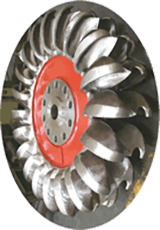fig 8 Wheel in workshop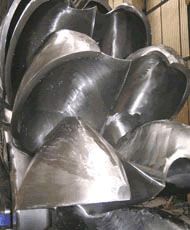fig 9 View detail of buckets

As it is well known, Pelton turbines work in presence of big heads, therefore also the conduits must be carefully checked and inspected by means of the usual quality investigation methods, in particular using the X ray to verify the welding of big thickness shells. The following figure shows in a schematic way the altimeter trend of the connection piping and subsequent penstock.

Just for curiosity, note the photographs (fig 11 and 12) of one wooden penstock with metal rings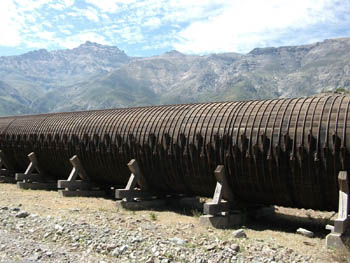fig 11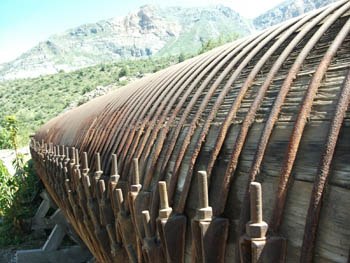fig 12

Fig 13 shows the wheel, which external diameter is of 4.4 m, with the respective shaft and in fig 14  note the six nozzles and, on the back, the spherical valve of the S. Giacomo sul Vomano (Riva Hydroart) powerplant with the following characteristics:

One of the most delicate component, easily subject to wearing, is the needle. Fig 15 shows one worn needle belonging to the turbine of Fig 16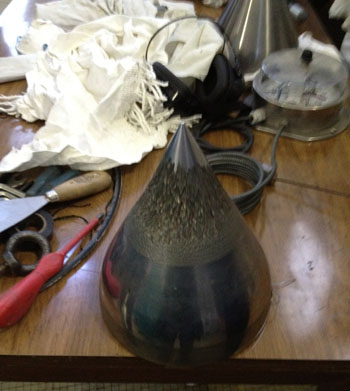fig 15 Pelton needle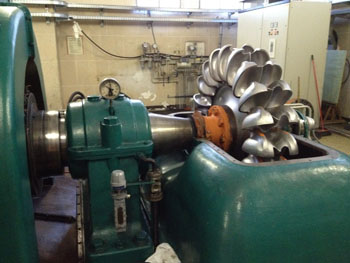fig 16 Pelton under maintenance

Fig 17 è shows horizontal Pelton turbine section, and in Fig 18 shows vertical Pelton turbine in workshop